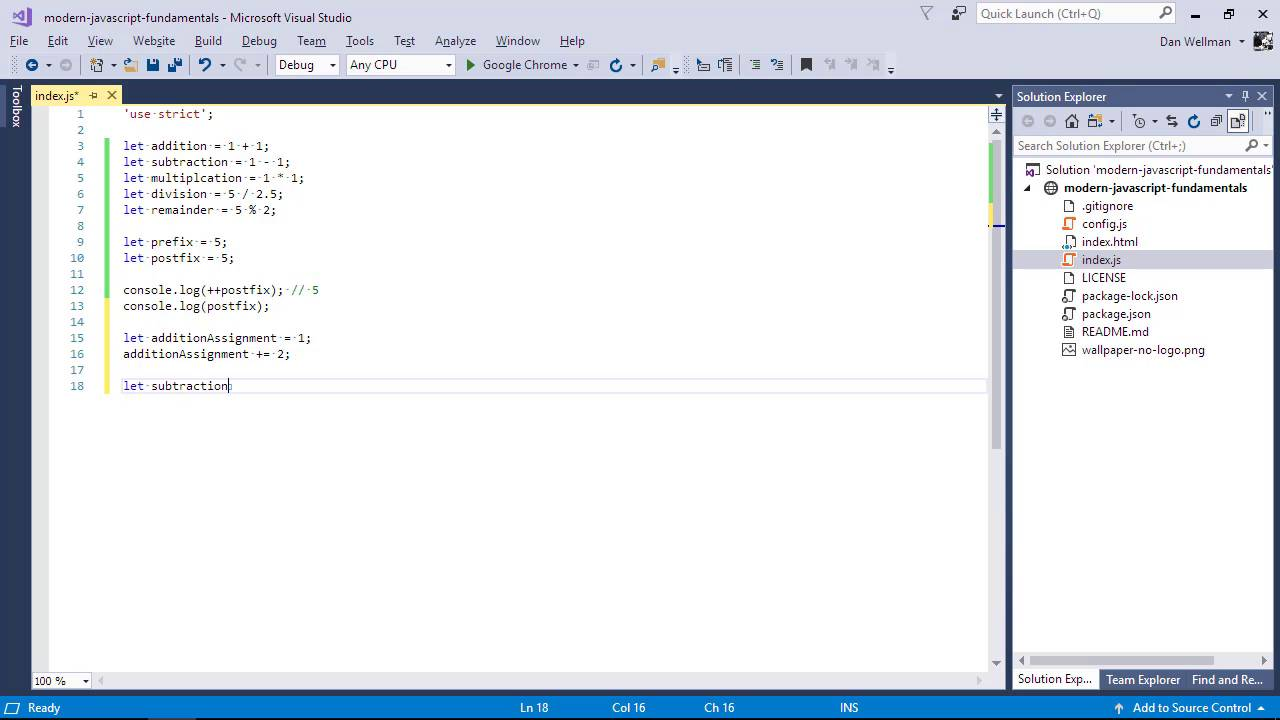Lessons: 65Length: 7.1 hours• Overview
• Transcript

# 2.3 Arithmetic, Assignment, and Comparison Operators

Special characters like + and - can be used in different combinations to perform operations on different things. Let’s see how some of the more basic operators, like arithmetic, assignment, and comparison operators behave.

Syntax

• +
• -
• *
• /
• %
• =
• <
• <=
• >
• >=
• **